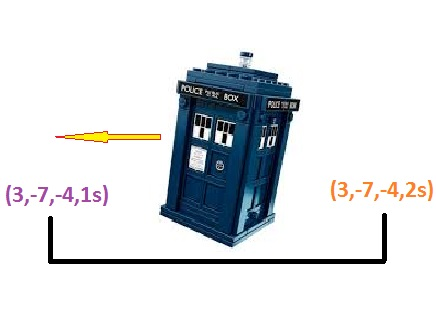# A 4 dimensional random walk!

Probability Level 5You start at the point $(x,y,z) = (1,1,1)$ in a Cartesian coordinate system, at time $t=0$. If we consider time to be a fourth dimension, we start at coordinate $(x,y,z,t) = (1,1,1,0)$

Every turn you take you move randomly in one of eight (yes 8!) possible directions, six of them are the six directions available to you on the Cartesian coordinate system ( $\pm \widehat{x}$, $\pm \widehat{y}$, $\pm \widehat{z}$ ), and the remaining two are to move backward or forward in time by one second.

What is the expected value for the number of moves (either in space or time or both) that it will take for you to land on a point $(3a, 3b, 3c, 3d$) where $a$, $b$, $c$ and $d$ are integers. $(a,b,c)$ is your $(x,y,z)$ location, and $d$ is how many seconds from when you started. Remember, time only moves (forward or backward) when you travel in time, otherwise it remains still.

Clarification: On any move you can go one unit in either direction on any of the three axes, or you can go backward or forward in time by one second each with probability $1/8$.

Note: If you end up moving in space, the move is instantaneous (i.e. time freezes), but if you move in time, you remain at a fixed location in space for that move.

Image credit: www.bbcamerica.com

×